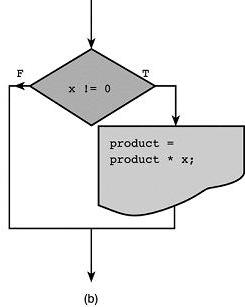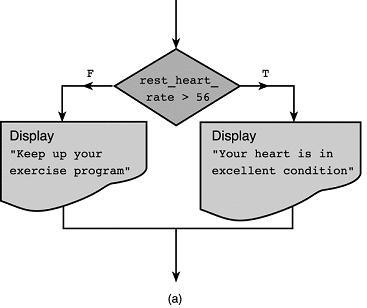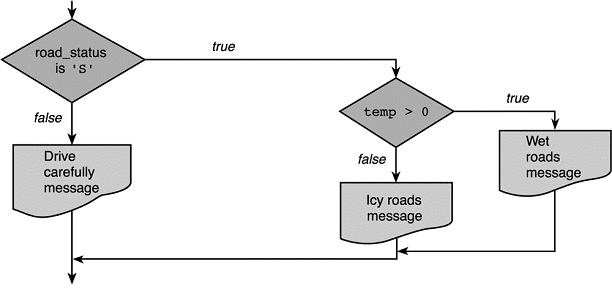## Forms of Selection Statements

 Single Alternative IF (divisor > 0) THEN     Quotient = Sum / Divisor END IF Double Alternative IF (job = manager) THEN     Compute Bonus as Salary * .05 ELSE     Computer Bonus as hourlyrate * 10 END IF Selection Sequence IF (number > 0) THEN     Increment positivecount END IF IF (number < 0) THEN    Increment negativecount END IF IF (number = 0) THEN    Increment zerocount END IF Nested Selection IF (temperature < 70) THEN     Print "Bring a Jacket" ELSE     IF (windspeed > 20) THEN         Print "Bring a kite"     ELSE         Print "Bring a frisbee     END IF END IF Multiple Alternative IF (score > 90) THEN    grade = 'A' ELSE IF (score > 80) THEN    grade = 'B' ELSE IF (score > 70) THEN    grade = 'C' ELSE    grade = 'F' END IFFlowcharts from Hanly & Koffman "Problem Solving and Program Design in C"